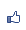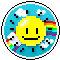唐老鴨之家 市長：燢 　副市長： 加入本城市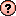｜推薦本城市｜加入我的最愛｜訂閱最新文章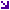本城市首頁討論區精華區投票區影像館推薦連結公告區訪客簿市政中心 (0)手動井字遊戲 瀏覽513｜回應0｜推薦0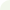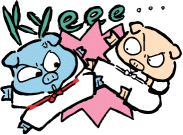燢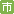等級：6 留言｜加入好友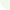Rem === 手動井字遊戲 ===

Rem === 兩個jpg圖檔(o.jpg和x.jpg)要放在同一目錄下

Dim turn As Boolean

Private Sub CommandButton1_Click()

Image1.Enabled = True

Image2.Enabled = True

Image3.Enabled = True

Image4.Enabled = True

Image5.Enabled = True

Image6.Enabled = True

Image7.Enabled = True

Image8.Enabled = True

Image9.Enabled = True

CommandButton2.Enabled = True

CommandButton2.Caption = "O 先下"

turn = True

End Sub

Private Sub commandbutton2_Click()

If turn Then

CommandButton2.Caption = "X 先下"

Else

CommandButton2.Caption = "O 先下"

End If

turn = Not turn

End Sub

Private Sub Image1_Click()

o = ThisWorkbook.Path & "\O.jpg"

X = ThisWorkbook.Path & "\X.jpg"

CommandButton2.Enabled = False

If turn Then

Else

End If

turn = Not turn

Image1.Enabled = False

End Sub

Private Sub Image2_Click()

o = ThisWorkbook.Path & "\O.jpg"

X = ThisWorkbook.Path & "\X.jpg"

CommandButton2.Enabled = False

If turn Then

Else

End If

turn = Not turn

Image2.Enabled = False

End Sub

Private Sub Image3_Click()

o = ThisWorkbook.Path & "\o.jpg"

X = ThisWorkbook.Path & "\x.jpg"

CommandButton2.Enabled = False

If turn Then

Else

End If

turn = Not turn

Image3.Enabled = False

End Sub

Private Sub Image4_Click()

o = ThisWorkbook.Path & "\o.jpg"

X = ThisWorkbook.Path & "\x.jpg"

CommandButton2.Enabled = False

If turn Then

Else

End If

turn = Not turn

Image4.Enabled = False

End Sub

Private Sub Image5_Click()

o = ThisWorkbook.Path & "\o.jpg"

X = ThisWorkbook.Path & "\x.jpg"

CommandButton2.Enabled = False

If turn Then

Else

End If

turn = Not turn

Image5.Enabled = False

End Sub

Private Sub Image6_Click()

o = ThisWorkbook.Path & "\o.jpg"

X = ThisWorkbook.Path & "\x.jpg"

CommandButton2.Enabled = False

If turn Then

Else

End If

turn = Not turn

Image6.Enabled = False

End Sub

Private Sub Image7_Click()

o = ThisWorkbook.Path & "\o.jpg"

X = ThisWorkbook.Path & "\x.jpg"

CommandButton2.Enabled = False

If turn Then

Else

End If

turn = Not turn

Image7.Enabled = False

End Sub

Private Sub Image8_Click()

o = ThisWorkbook.Path & "\o.jpg"

X = ThisWorkbook.Path & "\x.jpg"

CommandButton2.Enabled = False

If turn Then

Else

End If

turn = Not turn

Image8.Enabled = False

End Sub

Private Sub Image9_Click()

o = ThisWorkbook.Path & "\o.jpg"

X = ThisWorkbook.Path & "\x.jpg"

CommandButton2.Enabled = False

If turn Then

Else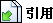引用網址：https://city.udn.com/forum/trackback.jsp?no=58536&aid=4896289